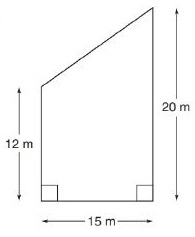Chapter 8.2, Problem 14E### Elementary Geometry for College St...

6th Edition
Daniel C. Alexander + 1 other
ISBN: 9781285195698

#### Solutions

Chapter
Section### Elementary Geometry for College St...

6th Edition
Daniel C. Alexander + 1 other
ISBN: 9781285195698
Textbook Problem
1 views

# In Exercises 13 to 18, find the area of the given polygon.To determine

To Find:

Explanation

Formula Used:

Split the quadrilateral into rectangle and triangle and find the corresponding areas and sum it up.

Area of rectangle =l×b

Area of right triangle =12×b×h

Calculation:

Let the vertices of the provided quadrilateral be A,B,C and D.

Let’s divide the quadrilateral into a rectangle and a triangle. Draw a perpendicular line from the point A to line CD and mark it as E.

Here ABCE forms a rectangle, the remaining AED forms the right triangle.

For the right triangle AED,

ED=CD-CE=CD-AB=20-12=8 m

### Still sussing out bartleby?

Check out a sample textbook solution.

See a sample solution

#### The Solution to Your Study Problems

Bartleby provides explanations to thousands of textbook problems written by our experts, many with advanced degrees!

Get Started

#### Evaluate the integral. 01(x10+10x)dx

Single Variable Calculus: Early Transcendentals

#### Simplify each expression. 24

Trigonometry (MindTap Course List)

#### Series Slate the definitions of convergent and divergent series.

Calculus: Early Transcendental Functions (MindTap Course List)